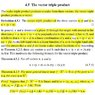# Linearity in the Vector triple product

• PcumP_Ravenclaw

#### PcumP_Ravenclaw

Dear all, I am trying to understand the vector triple product.
## x\times (y \times z) ##
As the vector triple product of x,y and z lies in the plane ## (y \times z) ## the vector ## x\times (y \times z) ## can be written as a linear combination of the vectors ## \pm y ## & ## \pm z##

In the passage attached, can anyone please explain this " As the left-hand side is a linear-function of x so is the right-hand side and this means that α and β are linear scalar valued functions of x"

Please show how the left-hand side is a linear function of x. What does linearity in vectors mean? Can anyone please explain in a bit of detail.

Also, what does Linear scalar valuesd functions of x mean? Please explain Theorem 4.2.5. To my understanding, this function changes a vector into a scalar. What does it mean to say ## f : ℝ^3 → ℝ ## is linear. Please give examples.

Danke..

#### Attachments

•VTP.jpg
65.5 KB · Views: 449
Please show how the left-hand side is a linear function of x. What does linearity in vectors mean? Can anyone please explain in a bit of detail.
If you multiply x by 5 (for example), the triple product gets multiplied by 5 as well. If you write x as (a+b) and calculate the triple-product with them individually, you can add the result to get the total result again.
This looks nicer when written as equations, but I'm sure you can find those in your textbook. Linearity is a very general concept, it does not matter if you have scalars, vectors or other mathematical objects.

•PcumP_Ravenclaw
In the equation ## X \times (Y \times Z) = (X \cdot a)Y + (X \cdot b)Z##. ## X ## is vector/cross multiplying ##(Y \times Z)## on the left-hand side but on the right-hand side it is scalar/dot multiplying so how is that possible. if it vector multiplies on the left it should also vector multiply on the right also, am I correct??

How do I relate theorem 4.2.5

## ƒ : ℝ^3 → ℝ ##, ## x \mapsto x \cdot a ##

with ## (X \cdot a)Y + (X \cdot b)Z ## ??

Danke..

Where does that equation come from and how is it related to 4.2.5?

The equation comes from the text I have attached. Theorem 4.2.5 is related to the equatino by linearity. I don't know how to relate them?? Maybe you can use examples to illustrate linearity.

That equation does not have a and b. For the left side, for example, x*z is a scalar, this scalar gets multiplied with the vector y and you have a vector again.